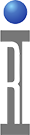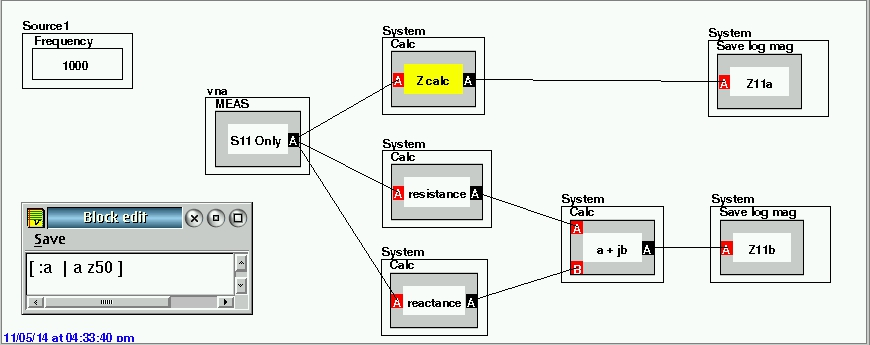Z-parameters are also known as open-circuit impedance parameters as they are calculated under open circuit conditions. i.e., Ix=0, where x=1,2 refer to input and output currents flowing through the ports (of a two-port network in this case) respectively.

The requirement to convert S11 to Z11 turns out to be very easy, since a one-port conversion of S11 into Z is the same as taking its "resistance" part and its "reactance" part and joining them as a single complex number. There are easy menu-driven calc blocks for resistance and reactance, plus a "hidden" calc that gives you both as a complex. Below is a sample test panel with both calculations shown. Create the yellow Calc button by modifying any one input calc block with a RMB click and select Edit Block....

The "block edit" shown is the text of the calculation block for "Z calc" in the top example (Z11a). The bottom example uses only standard calc blocks and available built-in calculations to obtain the same result (Z11b).https://roos.com/docs/RBEH-9QLSY8
ROOS INSTRUMENTS CONFIDENTIAL AND PROPRIETARY